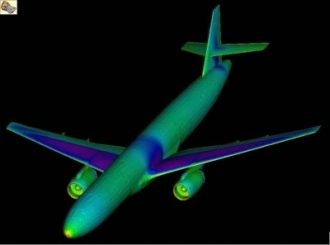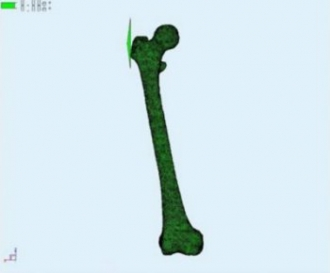# The Bloodhound Project Computational Fluid Dynamics and the BLOODHOUNDby Ben Evans, CFD Engineer & Aerodynamicist – BLOODHOUND aerodynamics

Most engineering fluid flow problems are now solved, at least in part, by using computational fluid dynamics (CFD). What this means is that we can use computers to help us solve the equations that govern fluid dynamics, rather than having to do them by hand.

### Which equations matter?

The governing equations for the majority of practical fluid flow problems are partial differential equations (PDEs) – in fact, that's the case for many naturally occurring phenomena. If you studied (or are studying) mathematics at A-level, you will probably have come across some simple differential equations and solved them analytically (using pen and paper).

The set of equations that are most relevant for describing the aerodynamic flows around BLOODHOUND are the so-called ‘Navier-Stokes’ equations. These are a set of five PDEs describing quantities such as the density, velocity and pressure of the airflow. (There's also a sixth equation if you want to model turbulence in the flowfield).

To get some idea of the degree of complexity of this set of equations, have a look at figure 1.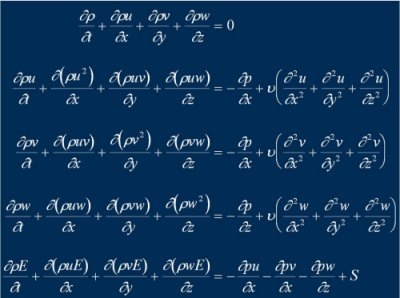Figure 1: The Navier-Stokes equations for viscous, compressible fluid flow

There is no hope of solving such a complex and coupled set of equations such as this by hand – even large supercomputers have to work hard to obtain solutions!

### The challenge in CFD

The development of CFD techniques has closely followed the development of numerical methods for solving partial differential equations. Numerical methods have been known since the time of Newton in the 1700s, but without the aid of the computer it was impossible to fully exploit these techniques.

Modern CFD has its roots in the 1950s with the advent of the digital computer. At the heart of all CFD numerical schemes is the fundamental question of how to represent a continuous function at a finite set of points (we call this 'discretisation'). In other words, how can we store a function defined for an infinite number of points (i.e. every possible position in space and time) in a finite way, and as accurately as possible?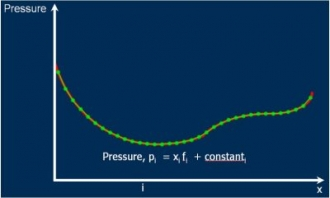Figure 2: Example of the discretisation / approximation of a pressure function

Here's an example. In figure 2 a pressure function has been 'discretised' so that the value of the function at a finite number of ‘nodes’ is stored and a linear interpolation of the solution is assumed between the nodes. The jump between each node is referred to as an 'element' and hence this kind of solution is often referred to as ‘the finite element method’.

### 3D solutions

The most popular method of achieving a three-dimensional finite element solution is to discretise the solution domain into a finite number of small cells or elements forming a mesh or grid, and to then apply a suitable algorithm to values stored at the intersections of the mesh (the nodes) to solve the governing equations – in our case, the Navier-Stokes equations.

The computational mesh can consist of elements of a whole variety of 3D shapes. For the CFD work carried out on BLOODHOUND, a mesh of hexahedra, prisms and tetrahedra numbering into the tens of millions was used!

### CFD and BLOODHOUND

The procedure for performing the CFD analysis on BLOODHOUND can best be described in the following stages:

1. The definition of the vehicle surface to be examined was provided to the team at Swansea University as a CAD (computer-aided-design) output from the design engineers based in the design office in Bristol. This geometry definition was then analysed via the FLITE3D computer system developed at the College of Engineering at Swansea University.

2. The CAD output was processed and a mesh generation computer program used to construct the computational mesh.

3. A ‘pre-processing’ computer program was then used to format the mesh in such a way that the Swansea University supercomputing cluster could be used to run the solver program. Over the course of the BLOODHOUND project we have also had computational support in the form of access to large supercomputers from Intel and HPC Wales.

4. The ‘equation solver’ program containing the Navier-Stokes solution algorithm was run on the supercomputing cluster.

5. A ‘post-processing’ software package was used to convert the solutions coming from the solver into meaningful flow visualisation plots and force distributions.

A selection of outputs from final visualisation stage is shown in figures 3 and 4. These visualisations helped the design team understand the behaviour of the car’s aerodynamics in terms of flow phenomena such as shock waves, boundary layers (the thin layer of slow-moving air close to the Car's surface) and pressure distributions. These pictures and force distributions were analysed and changes made to the design, and we then repeated steps 1 to 5 to check the outcomes of those changes.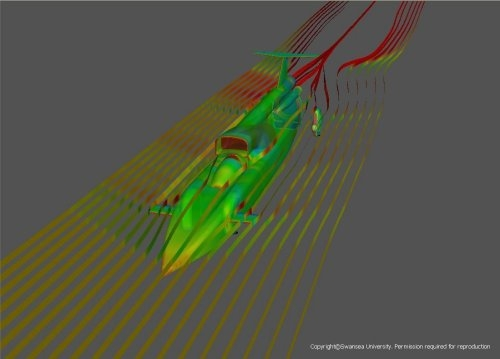Figure 3: Streamribbons and pressure contours over an intermediate BLOODHOUND configuration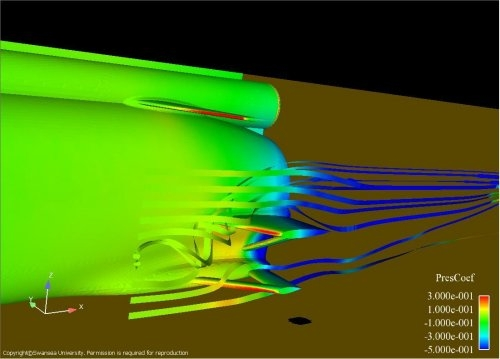Figure 4: Visualisation of a vortex being shed at the rear of BLOODHOUND impinging on the rear wheel struts

### Where else can we use CFD?

The applications for the computational modelling technologies being developed at Swansea are wide ranging, including: medicine; lightning strike modelling; building structural analysis; and even interstellar plasma flows. Essentially, any phenomena governed by partial differential equations can be simulated using the computational modelling approach of the finite element method.

In figure 6 you can see how biomedical modelling of stresses in a human femur is a phenomenon that can be described by a set of partial differential equations that can be modelled using the same techniques of finite elements that we used to study the aerodynamics of BLOODHOUND SSC.

Figures 5 and 6: Fluid-structure interaction modelling of a typical jet airliner; biomedical modelling of stresses in a human femur